# 【记录】继续更新excel公式去支持：小时的输入有2,3,4三种位数，字符串比较是否相等，当J列为空输出J EMPTY

Excel 2155浏览

【背景】

`=(\$H158- TIME(VALUE(MID(\$J158,1,1)),IF(LEN(\$J158)>1, VALUE(MID(\$J158,3,2)), 0)*60/100, 0))*24`

【已解决】excel中用公式实现单元格内容的条件判断：根据单元格内容长度执行不同的逻辑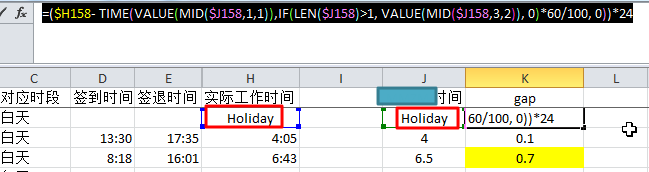【折腾过程】

1.所以需要：

`=IF(ISTEXT(\$H2),IF(\$H2=\$J2,0,1000),(\$H2-TIME(VALUE(MID(\$J2,1,1)),IF(LEN(\$J2)>1,VALUE(MID(\$J2,3,2)),0)*60/100,0))*24)`

• H2是字符串时：Holidy/Day off/sick等，去判断H2和J2是否相等
• 如果相等：值为0 -> 表示填写时间和实际时间无差别
• 如果不相等：值为1000, -> 此处随便设置为1000，表示填写不准确
• H2不是字符串时：
• 还是用之前的计算公式：
• (\$H2-TIME(VALUE(MID(\$J2,1,1)),IF(LEN(\$J2)>1,VALUE(MID(\$J2,3,2)),0)*60/100,0))*24
• 表示：
• 计算出时间差值，单位为小时。

2.不过，暂时还存在一个问题：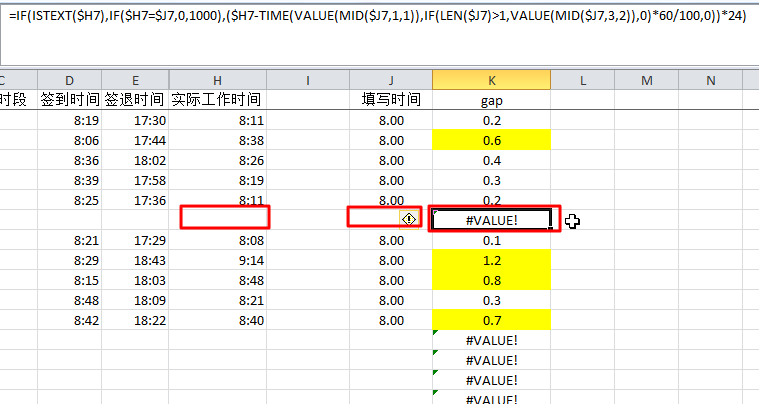H2和J2都是空值时，会计算失败，所以尝试去：

“excel 判断单元格是否为空,如果不为空数值加一”

 xxx <> ""

`=IF(\$J2<>"", IF(ISTEXT(\$H2),IF(\$H2=\$J2,0,1000),(\$H2-TIME(VALUE(MID(\$J2,1,1)),IF(LEN(\$J2)>1,VALUE(MID(\$J2,3,2)),0)*60/100,0))*24), "FOUND EMPTY")`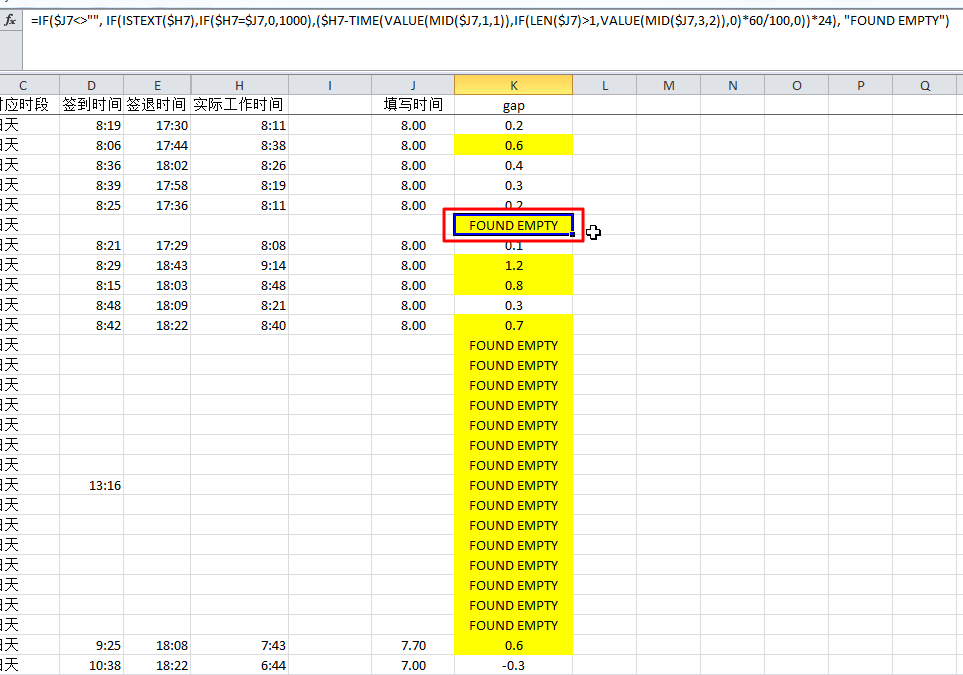4.后来又遇到一个bug：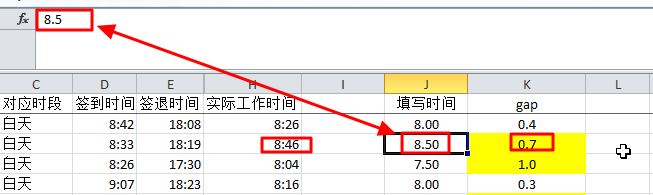J列，有三种可能：

8

8.2

8.25

=IF(\$J43<>"", IF(ISTEXT(\$H43),IF(\$H43=\$J43,0,1000),(\$H43-TIME(VALUE(MID(\$J43,1,1)),IF(LEN(\$J43)=1,0,IF(LEN(\$J43)=3,VALUE(MID(\$J43,3,1))*10, IF(LEN(\$J43)=4, VALUE(MID(\$J43,3,2)),0)))*60/100,0))*24), "J EMPTY")

`=IF(\$J43<>"", IF(ISTEXT(\$H43),IF(\$H43=\$J43,0,1000),(\$H43-TIME(VALUE(MID(\$J43,1,1)),IF(LEN(\$J43)=1,0,IF(LEN(\$J43)=3,VALUE(MID(\$J43,3,1))*10, IF(LEN(\$J43)=4, VALUE(MID(\$J43,3,2)),0)))*60/100,0))*24), "J EMPTY")`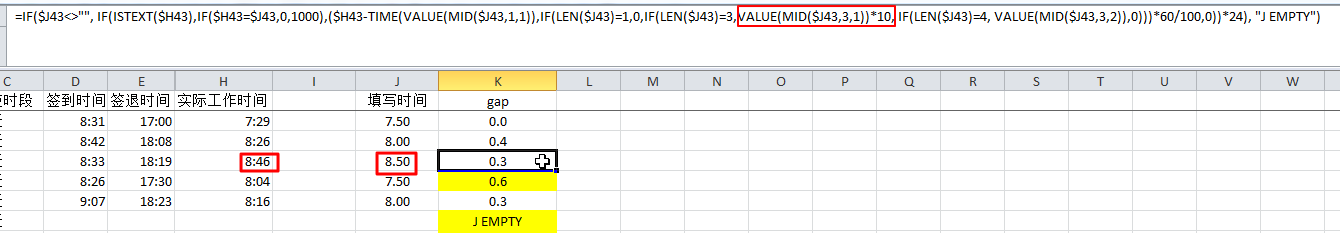【总结】

`=IF(\$J2<>"", IF(ISTEXT(\$H2),IF(\$H2=\$J2,0,"NOT SAME"),(\$H2-TIME(VALUE(MID(\$J2,1,1)),IF(LEN(\$J2)=1,0,IF(LEN(\$J2)=3,VALUE(MID(\$J2,3,1))*10, IF(LEN(\$J2)=4, VALUE(MID(\$J2,3,2)),0)))*60/100,0))*24), "J EMPTY")`

1.当J列的时间值，是，一位的（8），3位的（8.2），4位的（8.25），都可以正确计算出时间差值

2.当J列为空时，输出： J EMPTY

3.当H列和J列都是字符串时，判断两者字符串是否相等，如果相等，输出为0，否则输出为一个很大的数（比如1000），表示异常

83 queries in 0.173 seconds, using 22.34MB memory